# Bayesian Statistical Methods

## Partial solutions

### Chapter 2: From prior information to posterior inference

Jump to probem: 1, 3, 5, 7, 9, 11, 13, 15, 17

(1a) The posterior given in the chapter is $$\mu|Y \sim\mbox{Normal}\left(\frac{n}{n+m}{\bar Y},\frac{\sigma^2}{n+m}\right)$$ where $${\bar Y}=\sum_{i=1}^nY_i/n$$, so the 95% credible interval is $\frac{n}{n+m}{\bar Y} \pm 1.96\sigma/\sqrt{n+m}.$

(1b) If $$m\rightarrow 0$$ the 95% crebible interval converges to the classic z-interval and thus has frequentist coverage 0.95.

(3) The likelihood is $f(Y|\theta)=\theta\exp(-\theta Y)$ which is the kernel of a gamma distribution when viewed as a function of $$\theta$$. Therefore the conjugate prior is $$\theta\sim\mbox{Gamma}(a,b)$$. The resulting posterior is \begin{align} p(\theta|Y) &\propto f(Y|\theta)\pi(\theta)\\ &\propto [\theta\exp(-\theta Y)]\cdot[\theta^{a-1}\exp(-\theta b)]\\ &\propto \theta^{(a+1)-1}\exp[-\theta (Y+b)] \end{align} and $$\theta|Y\sim\mbox{Gamma}(a+1,Y+b)$$.

(5a) We need to select $$a$$ and $$b$$ so that the variance is 100 and median is 75. The variance is $$a/b^2$$ and so we must have $$a=100b^2$$. The median doesn't have a closed form so we simply search over a grid of $$b$$

  b_grid <- seq(0.1,1,0.0001)
med    <- qgamma(0.5,100*b_grid^2,b_grid)
b      <- b_grid[which.min(abs(med-75))]
a      <- 100*b^2
a

##  56.91194

  b

##  0.7544

  plot(b_grid,med); abline(v=b)  # Check graphically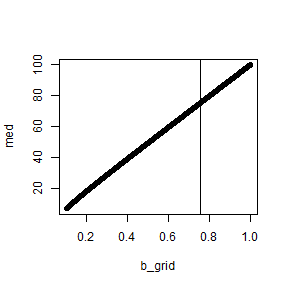lambda <- rgamma(10000000,a,b) # Check using MC sampling
hist(lambda,breaks=100)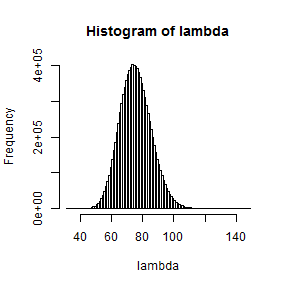var(lambda);mean(lambda>75)

##  99.89221

##  0.5000673


(7) Trial and error reveals that $$c=1.3$$ is a reasonable choice. This prior is actually somewhat informative for $$\alpha$$ and $$\beta$$, but gives an uninformative prior on the success probability.

  S     <- 1000000
c     <- 1.3
alpha <- rnorm(S,0,c)
beta  <- rnorm(S,0,c)
X     <- rnorm(S)
prob  <- 1/(1+exp(-alpha-X*beta))
hist(prob,breaks=50)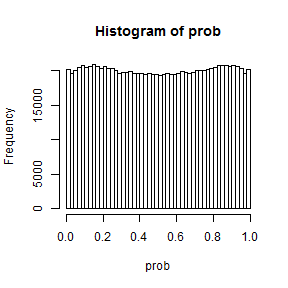(9a) A negative binomial assumes that each day is independent and has the same probability of a relapse, and that the experiment stops after a prespecified number of failures (in this case one), which describes the smoking cessation attempt fairly well. The main assumptions are independence and equal probability. Surely the probability varies with time since the beginning of the quit attempt, but it perhaps reasonable for a short study such as this.

(9b) This is approximated using Monte Carlo sampling (the plot is truncated at 14 because the PMF has a very heavy right tail):

  S     <- 100000
theta <- runif(S)
Y     <- rnbinom(S,1,theta)
plot(table(Y)/S,xlab="Number of days",ylab="Prior probability",xlim=c(0,14))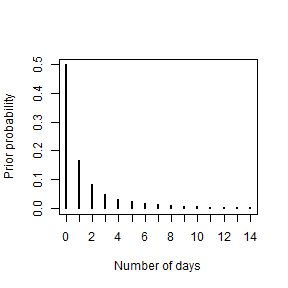mean(Y>=7);mean(Y>14)

##  0.12502

##  0.06283


(9c) The posterior is \begin{align} p(\theta|Y) &\propto f(Y|\theta)\pi(\theta)\\ &\propto [\theta^{1}(1-\theta)^Y]\\ &\propto \theta^{(2)-1}(1-\theta)^{(Y+1)-1} \end{align} and therefore $$\theta|Y\sim\mbox{Beta}(1+1,Y+1)$$, which is plotted below for $$Y=5$$

  S     <- 100000
theta <- rbeta(S,2,6)
hist(theta,breaks=50,xlab=expression(theta),ylab="Posterior")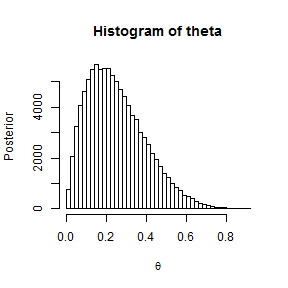(9d) The PPD is approximated using Monte Carlo sampling:

  S     <- 100000
theta <- rbeta(S,2,6)
Y     <- rnbinom(S,1,theta)
plot(table(Y)/S,xlab="Number of days",ylab="PPD",xlim=c(0,14))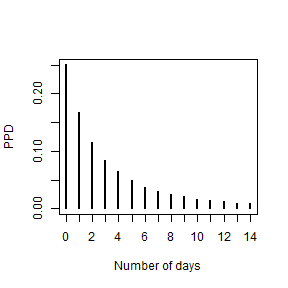mean(Y>=7)

##  0.22992


(11) The plots are below. I wouldn't say that any of the priors are uninformative for both variables, but the prior in (b) is the Jeffreys' prior so this is as close as you can get.

  S      <- 1000000
par(mfrow=c(1,2))

theta <- rbeta(S,1,1);
gamma <- theta/(1-theta); gamma <- gamma[gamma<100]
hist(theta,main="(11a)",breaks=50)
hist(gamma,main="(11a)",breaks=50)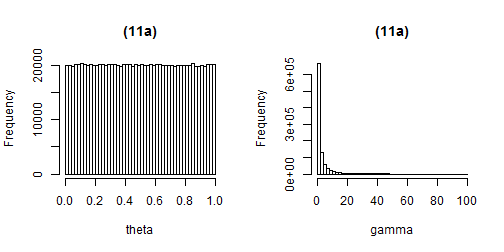theta <- rbeta(S,0.5,0.5)
gamma <- theta/(1-theta); gamma <- gamma[gamma<100]
hist(theta,main="(11b)",breaks=50)
hist(gamma,main="(11b)",breaks=50)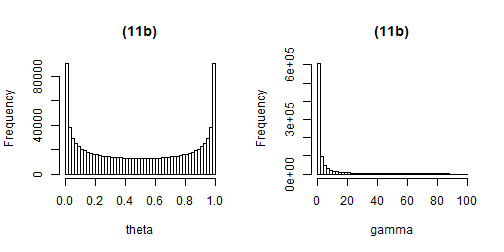gamma <- runif(S,0,100); gamma <- gamma[gamma<100]
theta <- gamma/(gamma+1)
hist(theta,main="(11c)",breaks=50)
hist(gamma,main="(11c)",breaks=50)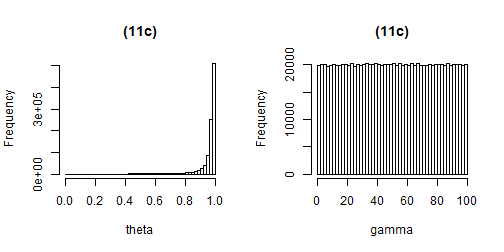gamma <- rgamma(S,1,1); gamma <- gamma[gamma<100]
theta <- gamma/(gamma+1)
hist(theta,main="(11d)",breaks=50)
hist(gamma,main="(11d)",breaks=50)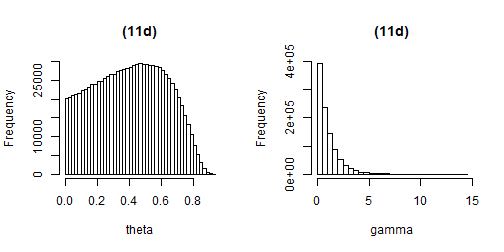par(mfrow=c(1,1))


(13) Denote the posterior as $$p(\theta|Y) = k f(Y|\theta)\pi(\theta)$$ for some constant $$k$$. By assumption $$p(\theta|Y) \ge kc(Y)\pi(\theta)$$ and thus $\int p(\theta|Y)d\theta \ge \int kc(Y)\pi(\theta)d\theta \ge kc(Y)\int\pi(\theta)d\theta = \infty$ since $$\pi$$ is an improper distribution.

(15a) The log likelihood and its first two derivatives are \begin{align} l(\lambda) & = -\lambda + Y\log(\lambda)\\ l'(\lambda) & = -1 + Y/\lambda\\ l'‘(\lambda) & =-Y/\lambda^2 \end{align} $$E(Y)=\lambda$$ and so the negative expected second derivative is $$1/\lambda$$ and the Jeffreys' prior is $$\pi(\lambda) = \sqrt{1/\lambda} = \lambda^{-½}$$.

  lambda <- seq(0.01,5,0.01)
plot(lambda,1/sqrt(lambda),type="l",ylab="Jeffreys' prior")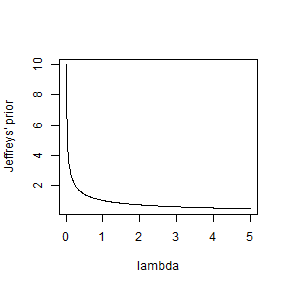(15b) The prior is not proper since $$\int_{0}^{\infty}\lambda^{-½}d\lambda = \infty$$.

(15c) The posterior is $p(\lambda|Y) = [\exp(-\lambda)\lambda^Y][\lambda^{-½}] = \lambda^{Y+½-1}\exp(-\lambda)$ and so $$\lambda|Y\sim\mbox{Gamma}(Y+½,1)$$ which is proper for all $$Y$$.

(17) The Jeffreys' prior from problem 15 is $$\pi(\lambda) = \lambda^{-½}$$ and the posterior is $$\lambda|Y\sim\mbox{Gamma}(Y+½,1)$$. The posterior is summarized below for $$Y=10$$:

  Y      <- 10
qgamma(0.5,Y+1/2,1)            # Posterior median

##  10.16861

  qgamma(c(0.025,0.975),Y+1/2,1) # Posterior 95% credible interval

##   5.141449 17.739438